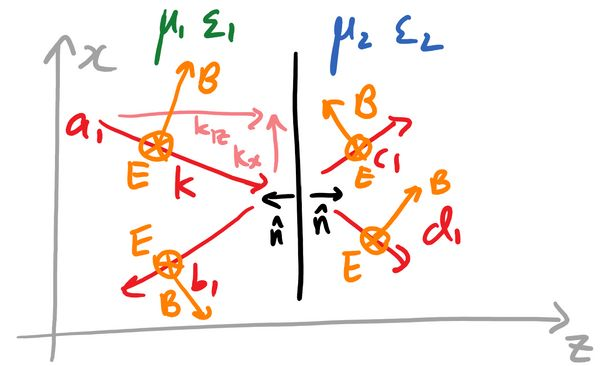Let's apply the material from the post on boundary conditions to calculate a transfer matrix. We will consider a TE wave travelling between materials with parameters $\mu_1,\epsilon_1$ and $\mu_2,\epsilon_2$:There are a few things to note:

1. This is a TE wave, so the electric field $\mathbf{E}$ is \emph{transverse} to the plane of incidence. In this case we chose to let $\mathbf{E}$ point into the page, which then determines the direction of the magnetic field since $(\mathbf{k},\mathbf{E},\mathbf{B})$ form a right-handed coordinate system.
2. The total wavevector $\mathbf{k}$ is made up of a component $k_z$ in the $z$-direction and $k_x$ in the $x$ direction.
3. The field on each side is made up of a forward-propagating wave ($a_1,c_1$) and a backward propagating wave ($b_1,d_1$). On the left we have

[eqTotalE]: $E=\left(a_1e^{i(k_{1z}z-\omega t)}+b_1e^{i(-k_{1z}z-\omega t)}\right)e^{ik_xx},$

and similarly for the right.

We want $(a_1,b_1)$ to represent the electric field in the first medium, but do we want them to represent $\mathbf{E}$ or $\mathbf{D}$? To decide, let's take a look at the boundary conditions:

$\hat{\mathbf{n}}\cdot\left(\mathbf{B}_2-\mathbf{B}_1\right)=0;; \hat{\mathbf{n}}\times\left(\mathbf{E}_1-\mathbf{E}_2\right)=0,$
$\hat{\mathbf{n}}\cdot\left(\mathbf{D}_2-\mathbf{D}_1\right)=0;;\hat{\mathbf{n}}\times\left(\mathbf{H}_2-\mathbf{H}_1\right)=0.$

The electric field is perpendicular to the boundary, so $\hat{\mathbf{n}}\cdot(\mathbf{D}_2-\mathbf{D}_1)=0$. On the other hand since $\mathbf{E}$ is perpendicular to $\hat{\mathbf{n}}$, you can convince yourself that the other boundary condition for the electric field gives $\mathbf{E}_1=\mathbf{E}_2$. Thus the electric field is continuous across the boundary, so we want $(a_1,b_1)$ to represent the electric field. In this case if $a_1,b_1$ are the fields just before the boundary and $c_1,d_1$ the fields just after, we have

$\mathbf{E}_1 = \mathbf{E}_2,$
$a_1+b_1 =c_1+d_1.$

Note that we have chosen coordinates such that the exponentials in Eq. [eqTotalE] are one right at the boundary.

Aside: If we had a TM wave we would choose $(a_1,b_1)$ to represent $\mathbf{H}$ rather than $\mathbf{B}$, since $\mathbf{H}$ is what would be continuous across the boundary and we would again have $a_1+b_1=c_1+d_1$. Note that this is just a convenience; you could use $\mathbf{B}$ if you really wanted to, and using $\mathbf{B}=\mu \mathbf{H}$ the boundary condition would then become $(a_1+b_1)/\mu_1=(c_1+d_1)/\mu_2$.

We need one more relation between the $a_1,b_1,c_1,d_1$, and we have two two boundary conditions we haven't used so far:

$\hat{\mathbf{n}}\cdot(\mathbf{B}_2-\mathbf{B}_1)=0;;\hat{\mathbf{n}}\times(\mathbf{H}_2-\mathbf{H}_1)=0.$

These are in terms of the magnetic field, which we want to relate to the electric field. This comes from Maxwell's equation:

$\nabla\times\mathbf{E}=-\partial_t\mathbf{B}=i\omega\mathbf{B}.$

What would happen if we took the $\mathbf{B}$ equation? Since $\mathbf{B}$ is being dotted with $\hat{\mathbf{n}}$, we see that the $z$-component of $\mathbf{B}$ is continuous across the boundary. This tells us that $(\nabla\times\mathbf{E})_z$ is continuous, which is:

$(\nabla\times\mathbf{E})_z=\frac{\partial E_y}{\partial x}-\frac{\partial E_x}{\partial y}=\frac{\partial E_y}{\partial x},$

since only $E_y$ is nonzero. Meanwhile the $\mathbf{H}$ equation gives us a relation for the $x$-component of $\mathbf{H}$, and

[eqCurlEx]: $(\nabla\times\mathbf{E})_x=\frac{\partial E_z}{\partial y}-\frac{\partial E_y}{\partial z}=-\frac{\partial E_y}{\partial z}.$

The wave is propagating in the $z$ direction, so this is what we want.

Using $\mathbf{B}=\mu\mathbf{H}$, Eq. [eqCurlEx] tells us that

$\left.\frac{1}{\mu_1}\frac{\partial E_y}{\partial z}\right\rvert_{Left}=\left.\frac{1}{\mu_2}\frac{\partial E_y}{\partial z}\right\rvert_{Right}.$

Looking at \cref{eqTotalE} the left hand side is

$\frac{ik_{1z}}{\mu_1}\left(a_1-b_1\right),$

while the right hand side will be

$\frac{ik_{2z}}{\mu_2}\left(c_1-d_1\right).$

Thus this boundary condition is:
[eqSecondBoundary]: $a_1-b_1=\frac{\mu_1}{\mu_2}\frac{k_{2z}}{k_{1z}}(c_1-d_1).$

The last thing is to write $k_{2z}/k_{1z}$ in terms of the parameters of the material. We know that $|k|=\omega\sqrt{\mu\epsilon}$, and $k_x^2+k_z^2=k^2$. Thus

$k_{1z} = \sqrt{\omega^2\mu_1\epsilon_1-k_x^2},$
$k_{2z} = \sqrt{\omega^2\mu_2\epsilon_2-k_x^2}.$

[eqSecondBoundary] thus gives us our second boundary equation:

$a_1-b_1=\frac{\mu_1}{\mu_2}\sqrt{\frac{\omega^2\mu_2\epsilon_2-k_x^2}{\omega^2\mu_1\epsilon_1-k_x^2}}(c_1-d_1).$

This is in terms of the frequency of the wave $\omega$, the parameters $\mu_i,\epsilon_i$ of the material, as well as $k_x$, which is a parameter telling us the angle of the input wave. For normal incidence we have $k_x=0$.